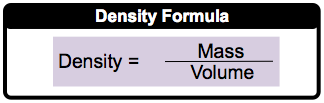Clutch Prep is now a part of Pearson
Ch.1 Matter and MeasurementsWorksheetSee all chapters

# Density

See all sections
Sections
What is Chemistry?
The Scientific Method
Classification of Matter
States of Matter
Physical & Chemical Changes
Chemical Properties
Physical Properties
Intensive vs. Extensive Properties
Temperature (Simplified)
Scientific Notation
SI Units (Simplified)
Metric Prefixes
Significant Figures (Simplified)
Significant Figures: Precision in Measurements
Significant Figures: In Calculations
Conversion Factors (Simplified)
Dimensional Analysis
Density
Specific Gravity
Density of Geometric Objects
Density of Non-Geometric Objects

Density represents the mass of an object or compound within a given volume.

###### Understanding Density

Concept #1: DensityExample #1: If the density of an unknown metal is 21.4 g/cm3, express its density in lb/ft3.

Practice: When lead levels in blood exceed 0.80 ppm (parts per million) the level is considered dangerous. 0.80 ppm means that 1 million g of blood would contain 0.80 g of Pb. Given that the density of blood is 1.060 kg/cm3, how many grams of Pb would be found in 400.00 mL of blood at 0.620 ppm?